﻿ Adsorption Isotherms and Kinetics of Ni(II) and Pb(II) Ions on New Layered Double Hydroxides-Nitrilotriacetate Composite in Aqueous Mediap-ISSN: 2163-2839    e-ISSN: 2163-2847

2016;  6(1): 17-33

doi:10.5923/j.aac.20160601.03### Adsorption Isotherms and Kinetics of Ni(II) and Pb(II) Ions on New Layered Double Hydroxides-Nitrilotriacetate Composite in Aqueous Media

Rasheed M. A. Q. Jamhour 1, Taher S. Ababneh 1, Albara I. Al-Rawashdeh 1, Ghassab M. Al-Mazaideh 1, Tareq M. A. Al Shboul 1, Taghreed M. A. Jazzazi 2

1Department of Chemistry and Chemical Technology, Tafila Technical University, Tafila, Jordan

2Department of Chemistry, Faculty of Science, Yarmouk University, Irbid, Jordan

Correspondence to: Rasheed M. A. Q. Jamhour , Department of Chemistry and Chemical Technology, Tafila Technical University, Tafila, Jordan.
 Email: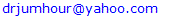Abstract

Anionic layered double hydroxides (LDH) known to have high adsorption capacities and are easily synthesized in the laboratory. With nitrilotriacetate (NTA) anions inclusion as ligand, which is incorporated as interlayer anion in the LDH structure via ion-exchange or coprecipitation reaction, this inclusion would give the Zn-Al LDH material considerable potential as chelate for metal cation contaminants in the aqueous environment by changing the regular behavior of anion exchange to cationic exchanger or serpent. The characterization of the new sorbent is carried out by FTIR spectroscopy (FT-IR), powder x-ray diffraction and scanning electron microscope (SEM). The potential capacity of uptake of Ni(II) and Pb(II) at pH of 5 is attributed to the complex formation between metal ions and NTA in the interlayer of LDH as well as surface adsorption. This work aimed to investigate the kinetics of the sorption mechanism using batch technique at different temperature, pH and various metal concentrations. In addition to the kinetic study of adsorption isotherms and the interaction of adsorbent-adsorbate time as parameters, using a pseudo-first-order, pseudo-second-order and intra-particle diffusion kinetic models. The results show better correspondence to a pseudo-second-order kinetics model with high correlation coefficients (R2= 0.997 for the initial concentrations). The model of Freundlich is appropriate to describe the experimental adsorption results with sorption capacities of 7.153, 6.807 mg/g for Ni(II) and Pb(II) respectively. These behaviors are attributed to the structural construction of the Zn-Al-NTA material. All these results have shown the high efficiency of Zn-Al-NTA adsorbent for the fast removal of Ni(II) and Pb(II) from aqueous solution.

Cite this paper: Rasheed M. A. Q. Jamhour , Taher S. Ababneh , Albara I. Al-Rawashdeh , Ghassab M. Al-Mazaideh , Tareq M. A. Al Shboul , Taghreed M. A. Jazzazi , Adsorption Isotherms and Kinetics of Ni(II) and Pb(II) Ions on New Layered Double Hydroxides-Nitrilotriacetate Composite in Aqueous Media, Advances in Analytical Chemistry, Vol. 6 No. 1, 2016, pp. 17-33. doi: 10.5923/j.aac.20160601.03.

### 1. Introduction

Application of nanoparticles for the removal of contaminates has become an interesting area of research. The unique properties of nanomaterials are providing opportunities for the removal of metals in highly efficient ways and thus have been studied by many researchers [1-4]. Nanoparticles of Layered double hydroxides LDH or anionic clays have been of interest to researchers in many fields of technology and science due to their unique properties and various applications especially as adsorbents for the removal of pollutants , ion-exchangers , catalyst precursors  and more recently in pharmaceutics for drug release control [8-10]. In adsorption technology, LDH have already been characterized by many properties that showed to have high adsorption capacity due to its high specific surface area, mechanical and chemical stability, high exchange capacity, negative surface charge and structural variability . LDH are natural minerals and synthetic materials that consist of layered structure with positive charge brucite-type (Mg(OH)2) layers of mixed metal hydroxides. In which, magnesium atoms coordinate with hydroxyl groups in an octahedral geometry resulting in layers that interact with each other by weak forces. Exchangeable anions are located in the interlayer spaces in order to balance the positive charge of the layers. LDH have a general formulation of [M+2 1-x M+3 x (OH)2]+x (Am-)x/m.nH2O, where M+3 and M+2 represent metal atoms arranged octahedrally within the structure and Am- represents a general anion such as Cl-, OH-, CO3 -2, etc. Trivalent metal atoms have substituted the divalent metal atoms, which generates positively charged layers [12, 13]. The interlayer region also contains water molecules, the amount of water is determined by factors such as the nature of the interlayer anions, the water vapor pressure and temperature. The water molecules are attached to both the metal hydroxide layers and interlayer anions through extensive hydrogen bonding . The generation of an organic-inorganic hybrid material is accomplished by incorporating an organic anion in the interlayer space of the layered inorganic matrix by a typical ion-exchange mechanism between the anion sited, which is free to move, and the organic anion. It is also possible to exchange not only organic but also inorganic anions, as well as complexes at variable oxidation states [15-17]. The layer surfaces of LDH are structurally similar to Kaolinite (Al2Si2O5), in which it is a dioctahedral layered aluminosilicate, consists of aluminum atoms coordinated octahedrally with oxygen atoms and hydroxyl groups on the outer surface of the layer. While the inside surface resembles the structure of silica, where silicon atoms are coordinated tetrahedrally with only oxygen atoms. Therefore, less hydroxyl groups are found when compared by LDH layers that are decorated with hydroxyl groups at both layer surfaces [18, 19]. This fact makes the LDH to be susceptible to the same type of reactions with Kaolinite and probably with higher reactivity, due to the availability of reaction sites. Therefore, many chemical modifications using the host - guest system for incorporating molecules in the interlayer space were found in the literature, for instance, different anionic dyes were incorporated into Zn-Al- LDH , tetraphthalate, naphthalene-2,6-disulfonate, and anthraquinone-2,6-disulfonate anions were also incorporated with the same type LDH , and different carboxylate anions with Zn-Al-LDH were synthesized . In most cases the intercalated molecules are often present as neutral molecules or anions.
The aim of this study is to incorporate nitrilotriacetate anion in the interlayer space of Zn-Al-LDH by a direct conventional coprecipitation method, which is expected to give a new avenue to a number of applications especially in the industrial processes. In this work we focus on Ni(II) and Pb(II) heavy metal ions which have become a serious environmental issue, especially due to their toxicity and tendency to bioaccumulation . Ni(II) and Pb(II) released to the environment through industrial effluents have been found toxic and responsible for serious pollution problems and adverse effects against human health including: kidneys damage, nervous and reproductive systems attack as reported by Lin and Juang . It is necessary that such heavy metal must be removed before the industrial effluents transferred to the environment. Several methods are used for treatment of heavy metal aqueous solutions, such as ion exchange, precipitation, phytoextraction, ultrafiltration, reverse osmosis, electrochemical deposition, adsorption using activated carbons or zeolites [25, 26]. Because of the high cost of above the processes, recourse of low-cost adsorbents has been appeared to be an alternative opportunity in adsorption technology and interest towards these materials is growing . LDH found its way in low cost, ease of preparation and most importantly in its selectivity when incorporated with ligand of interest.

### 2. Experimental

#### 2.1. Materials and Methods

The starting materials Zn(NO3)2·6H2O, Al(NO3)3·9H2O, 50% aqueous NaOH, and aqueous solutions of nitrilotriacetate were prepared fresh prior to use. NTA were obtained from commercial sources, analytical grade, and used as received. The analysis and characterization was carried out by using: Powder X-ray diffraction (PXRD) data were collected at room temperature on a Philips X'pert 3065 diffractometer with a curved graphite monochromator (Cu-Kα radiation) in a Bragg–Brentano para-focusing optics configuration. Synthesized samples were scanned in 0.02°- 2θ steps with a counting time of 1s per step. A Mettler. FTIR spectra were obtained with KBr pellets using a Mattson Infinity FTIR 60 AR infrared spectrophotometer. These analyses were carried out at the Institute for Inorganic Chemistry, Friedrich-Alexander University, Erlangen-Nürnberg, Germany. The scanning electron microscopic images we carried out at the Yarmouk University –Jordan using EDX (JEOL JED-2300) SEM.

#### 2.2. Preparation of Zn-Al-NO3 Host Material

The initial LDHs, [Zn-Al-NO3], used in the ion-exchange intercalation reactions, was prepared according to our previous reported procedure . An 18.8 g of Zn(NO3)2.6H2O and 11.7 g of Al(NO3)3·9H2O were dissolved in 80 mL of deionised decarbonated water(DDW) to give a total metal Zn: Al ratio of about 2. This solution was then added drop by drop to another solution containing 6.25 g NaOH and 9.1 g NaNO3 in 45 mL of double distilled water. The mixture was stirred during the precipitation reaction at the 60°C for two hours. The pH was maintained until around 8-9 by adding NaOH solution. The resulting gel or slurry like precipitate was digested at 60°C for 24 h. This slurry was filtered and washed with hot DDW, followed by the addition of a small amount of acetone to facilitate drying, and kept in drying oven for 16 h at 60°C.

#### 2.3. Preparation of Zn-Al-NTA Intercalation Compound

Typical intercalation experiments were conducted by two routes first by anion exchange according to the following procedure: 5 g of the [Zn-Al-NO3] precursor was suspended in 0.5 L of a 0.01 M aqueous solution of NTA. The NTA solution was prepared by mixing solutions of NTA and NaOH, with an acid / base ratio varying from 1: 2 to 1: 5 to give pH of about 7. The suspension was kept on a slow stirring for 12 h at 65°C for ion-exchange procedure. The product was filtered and air dried at room temperature. FT-IR spectroscopy was used to check the completeness of anion exchange. The NTA-intercalated LDHs were prepared by the second route of direct mixing or coprecipitation method as described above and reported earlier . Typically, the solution of Zn(NO3)2·6H2O (1.97 g) and Al(NO3)3·9H2O (1.12 g) in DDW (100 mL) was prepared. Another aqueous solution of 0.1 M nitrilotriacetate was prepared with NaOH (0.5 g) in (50 mL). The NaOH solution was added before hand to dissolve the acidic form of NTA and to adjust the solution to a neutral pH. Maintaining the pH in the range 8–10, the resulting gel-like slurry was digested overnight at 70°C, filtered and washed several times with DDW and dried in an oven at 60°C for 16 h. Calcination was carried out at 450°C a muffle furnace.

All adsorption experiments were carried out with accurately weighted adsorbent samples of LDH-nitrilotriacetate composites (0.20 g) in 30 ml of standard Ni(II) and Pb(II) metal ion solutions at 25°C. The mixture is thoroughly shaken in a capped container using a magnetic stirrer operating at 200 rpm for up to 120 mins. Samples were then centrifuged at 4000 rpm and the supernatant liquid was separated from the solids for analysis. The concentration of residual Ni(II) and Pb(II) metal ions was determined using atomic absorption measurements, thus enabling evaluation of metal adsorption capacities of LDH-nitrilotriacetate composite adsorbents. The optimum adsorption conditions were obtained by investigating the adsorption efficiency of different solution conditions including: the effect of contact time, pH value and initial concentration of metal ion (Co). The efficiency of each adsorption process is expressed in terms of removal percentage of metal ion from solution, and is defined as the ratio of the difference in the adsorbate concentration before and after adsorption (CoCe), to the initial concentration of the metal ion in the aqueous solution (Ce) as shown in the following equation: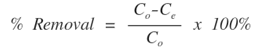The adsorption efficiency of the LDH-nitrilotriacetate composite is discussed and compared according to using Ni(II) and Pb(II) metal adsorbates.

### 3. Results and Discussions

#### 3.1. Characterization of Zn-Al -NTA Composite

FT-IR spectrum analysis of the Zn-Al- LDH composite shows the characteristic identity of the host LDH and after intercalation of NTA. In the parent LDH spectra Fig.1A, hydroxyl groups were identified by the characteristic broad peak assigned to the stretching vibrations of O-H bonds in the range 3750–3250 cm-1 Fig.1B, while the sharp bands at ~1615 and ~1384 in the spectra of the intercalated LDH attributed to stretching vibrations of -COO bonds of asymmetric and symmetric carboxylate anion. A peak was observed at about 742 cm−1 for the symmetric stretching mode ν1 of NO3 and signal at 1384 cm-1 is associated with the stretching vibrations of C-OH groups. This band is absent in the precursor ([Zn2Al(OH)6]NO3.xH2O) intercalated material. Indicating a complete exchange reaction with NTA. These two absorption bands actually confirm the presence of the intercalated functional groups of nitrilotriacetate . Whereas, the strong band at higher frequency ~1650 cm-1 corresponding to COOH stretching vibration. The presence of such diversity of functional groups with more -OH on the surface and amino groups of the NTA molecule makes it an attractive material for adsorbing cationic species. The coordination of the carboxylate groups of the NTA ligand to the metal ion was also suggested in our previous study , by the red-shift or lower frequency shift of about ~15 cm-1 in the wave number between νas and νs of (–COO) Fig.1C. This shift might be due to possible interaction, which could be affected by the H-bonding between the intercalated NTA anion and the hydroxyl groups of the brucite layers. Below 850 cm−1, the FTIR spectra of the three samples contain same bands arising from the host lattice of LDH. The C-N and the C-H stretching vibrations are also observed at ~1105 and ~3430 cm-1, respectively. A schematic presentation of the possible mechanism of intercalation of NTA anion is presented by simple ion-exchange reaction with NO3 shown in scheme 1.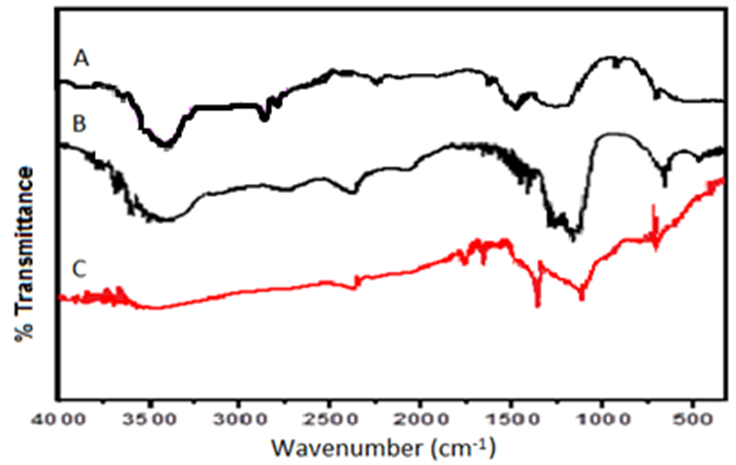Figure 1. FTIR spectra of: A) The synthesized Zn-Al –NO3 host material, B) After intercalation with NTA guest anion and C) after adsorption of metal ion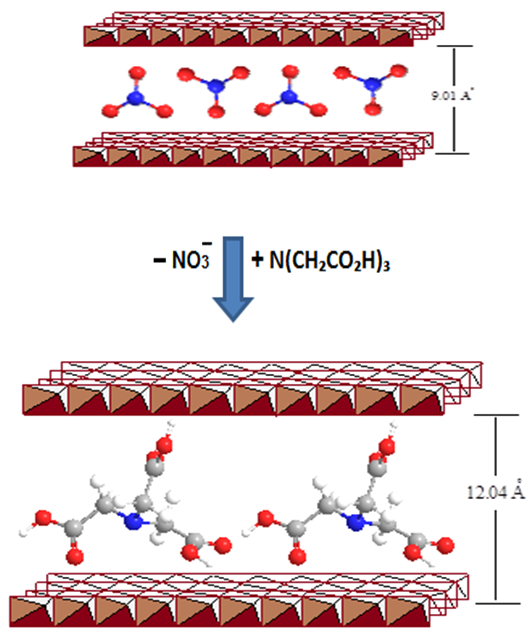Scheme 1. Representation of the exchange reaction of NTA with NO3 anions
The powder XRD patterns of Zn-Al-NO3 shown in Fig. 2, is typical of a layered LDH material, which are usually indexed on the basis of a hexagonal unit cell which is in agreement with the PXRD pattern of those reported in literature . Furthermore, it is well known that there is a direct correlation between the lattice parameter (a) of the unit cell (2θ degree) and d-spacing of different reflections within the LDH sheets. The (003) reflections are observed up to 5.7° 2θ. The basal spacing d003 shifted from about 9.01 Å for Zn-Al-NO3 (Fig. 2A) to 12.04 Å for the pillared Zn-Al-NTA (Fig. 2B). Assuming a thickness of ca. 4.8 Å for the double hydroxide layer, these results indicate that the gallery height generated in the hybrid materials is 7.24 Å. The (006) peaks which are shifted to lower 2θ values indicating intercalation of planar NTA anions oriented parallel to the metal hydroxide sheets. The PXRD pattern for the precursor material also contains small reflections above ca. 2θ = 25°. The d012, d015, and d016 indicating relatively large crystallite size, and good defined reflections, giving the layer reasonable stacking as it can also be seen from the SEM images.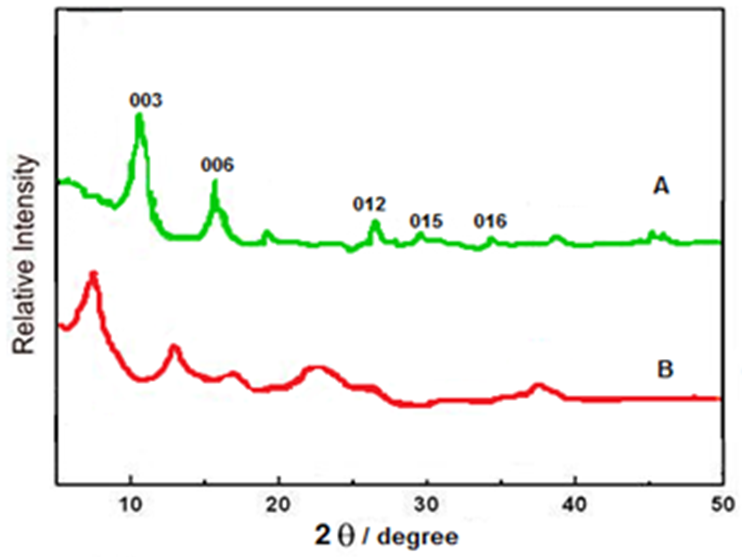Figure 2. PXRD pattern of Zn-Al LDHs: A) Zn-Al (NO3) and B) Zn-Al (NTA) phase
SEM images (Figure 3) show that the LDH as prepared, after calcination and with metal ion adsorption exhibited regular belt-like structures. After intercalation of NTA molecule no phase separation was observed, which implies the formation of complete planar sheets in the inner structure consisting of probably crosslinking acetic acid groups of the NTA molecule. The host Zn-AL-NO3 exhibited a very clearly defined belt structure (Fig. 3a). The reason is that there are numerous acetate anions (–COO) in between the layers as well as on the surface. The electrostatic repulsions among the acetate anions can result in the expansion of the LDH network and the increase in the size of the gallery height. Therefore, metal ions can easily diffuse in and adhere to the LDH-NTA main structure. The observed melt-like pellets, on the other hand, indicate the effect of calcination (Fig. 3b) which is also similar to that due to metal ion adsorption. In which, adsorption of metal ion weakens the electrostatic repulsion between the acetic acid groups that might lead to shrinkage of belts and reduce the porosity (Fig. 3c). In similar way high temperature of 450°C would lead to hydroxylation and subsequent removal of NTA which gave a collapsed layer structure of metal oxides.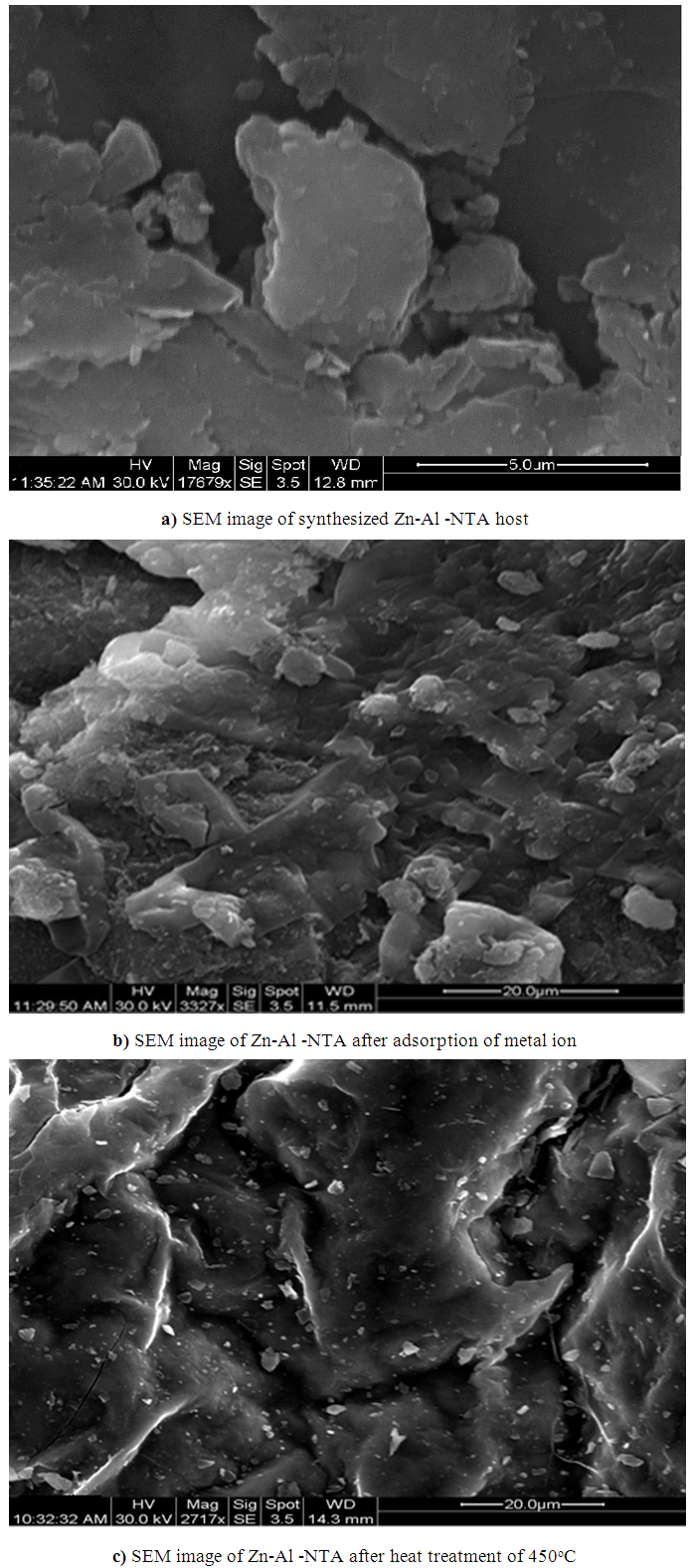Figure 3. SEM images of synthesized nanoparticles of (a) Zn-Al -NTA host (b) Zn-Al -NTA after adsorption of metal ion and (c) after heat treatment at 450°C
 Table 1. Conditions applied for the adsorption of nickel(II) and lead(II) ions by Zn-Al -NTA material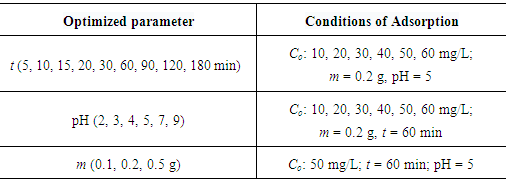#### 3.2. Effect of Contact Time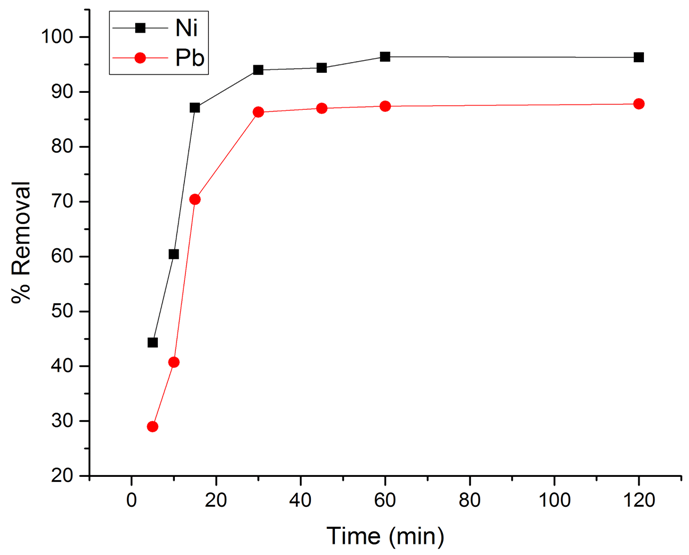Figure 4. Effect of different contact times on percentage removal of the metal ions

#### 3.3. Effect of pH

The effect of solution pH (2 to 10) on the % removal of Ni(II) and Pb(II) ions on LDH-nitrilotriacetate composites is shown in Figure 5. As can be seen from the figure, the adsorption process is pH dependent with the highest percent of Ni(II) and Pb(II) removal at pH 5, at which the percent removal of Ni(II) and Pb(II) is 96.0% and 86.3%, respectively. The solution pH is known to be an important variable affecting the amount of ion adsorption by an adsorbent. It can influence the protonation of the functional groups on the adsorbents as well as the solution chemistry of the heavy metal ions . The uptake of Ni(II) and Pb(II) was found to increase sharply when the pH value was increased from 2.0 to 3.0, beyond which values a gradual increase in the adsorption capacity was observed. The hydroxyl and acetate groups in the Zn-Al-NTA composite are primarily responsible for the specific binding of metal ions. At low pH it is difficult for metal ion to find available sites for interaction as acetate groups are present in the form of COOH rather than –COO. This situation conversed at higher pH value whereby more ionized acetate groups were available, thereby, increasing the interaction between both acetate and/or hydroxide groups and the metal ions, causing an increase in Ni(II) and Pb(II) adsorption. For adequate recovery and to avoid the formation of metal hydroxide precipitate at higher pH, the subsequent adsorption experiments were carried out at pH 5.0.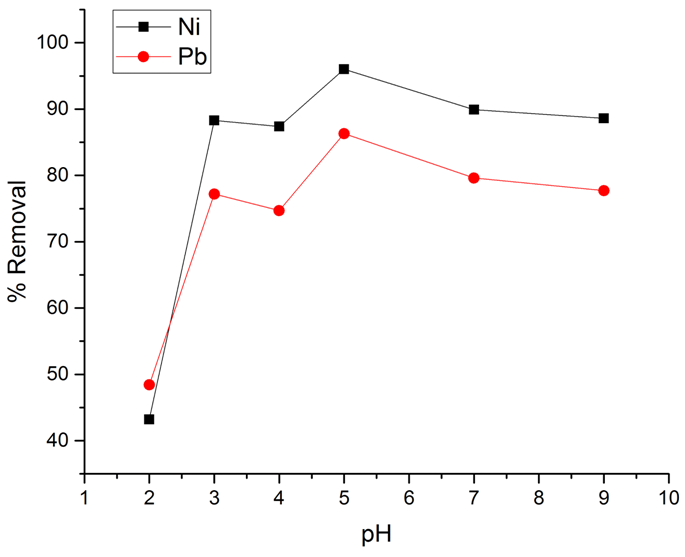Figure 5. Percentage removal of the metal ions at different pHs

#### 3.4. Effect of Metal Ion Initial Concentration

Initial ion concentration plays an important role in determining the amount of metal ion adsorption by an adsorbent. In this study the adsorption amounts of Ni(II) and Pb(II) metal ions on Zn-Al-NTA were measured at different ion concentrations (10 to 60 ppm). The result in (Figure 6 a) shows that the adsorption amount increased linearly with the initial ion concentration from 10 to 60 mg/L. When the Co was 10 mg/L, the qe reached 1.370 mg/g for Ni(II) and 1.236 mg/g for Pb(II), with % removal of 91.4 and 82.4, respectively. By increasing the initial metal ion concentration to 50 mg/L, the qe reached 7.153 mg/g for Ni(II) and 6.807 mg/g for Pb(II), with % removal of 95.4 and 90.8, respectively (Figure 6 b). This demonstrates that the Zn-Al-NTA composite have an excellent capacity for removing Ni(II) and Pb(II) from aqueous media. The equilibrium adsorption isotherm is fundamental in describing the amount of metal ion adsorbed onto the solid adsorbent and the concentration of dissolved metal ions at equilibrium. The present adsorption isotherm data were evaluated by means of the Langmuir, Freundlich and Temkin adsorption isotherm models.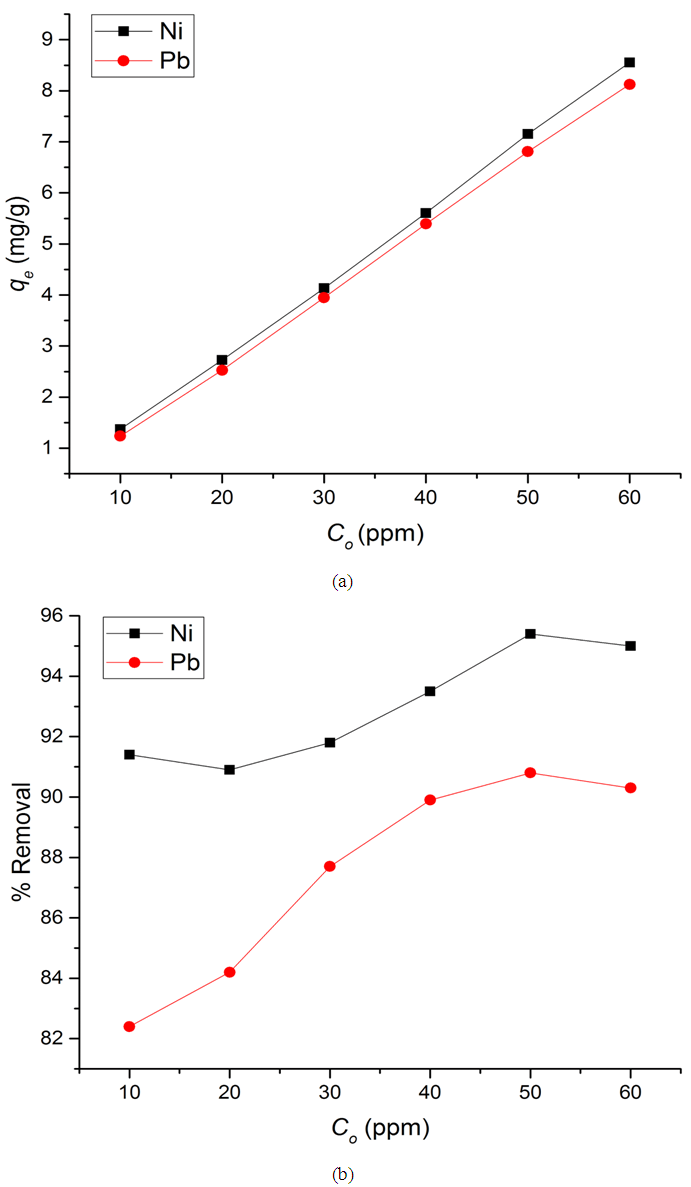Figure 6. (a) Amount of metal ions adsorbed per unit mass of Zn-Al-NTA (mg/g) at a different adsorbate concentration and (b) Percentage removal of metal ions at different adsorbate concentration

The adsorption isotherms were primarily used to investigate the amounts of nickel and lead metal ions sorbed by a certain mass of Zn-Al-NTA. We have used different isotherm models to find the position of equilibrium of metal ions between solution and Zn-Al-NTA mass. Among the most common isotherms are the monolayer adsorption model developed by Langmuir and the multilayer adsorption Freundlich model.
##### 3.5.1. Langmuir Isotherm
Langmuir model is based on monolayer adsorption coverage of solute particles into a finite number of identical sites on the surface of the sorbent . This model assumes that adsorbed molecules cannot move across the surface or interact with each other. The linear form of Langmuir model is expressed as follows: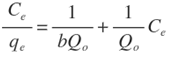Where,
Ce is the equilibrium metal ion concentration in solution (mg/L);
b is the Langmuir affinity constant (L/mg);
Qo is the adsorption capacity at equilibrium (mg/g).
qe is the amount of metal ions adsorbed per unit mass of Zn-Al-NTA adsorbent (mg/g) and can be calculated using the following relation: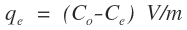Where,
Co is the initial metal ion concentration in solution (mg/L);
V is the volume of the solution (L);
m is the mass of the Zn-Al-NTA adsorbent (g);
(Co-Ce) represents the adsorbed amount of metal ions.
If experimental data are best described by Langmuir isotherm, a plot of (Ce/qe) versus (Ce) will give a straight line with slope of (1/Qo) and intercept of (1/bQo). Such plot is used to find the Langmuir parameters. Langmuir plots for the adsorption of (a) Ni(II) and (b) Pb(II) on Zn-Al-NTA adsorbent is depicted in Figure 7, it is clear from the deviation of the graph from linearity and the lower correlation coefficient values R2 (0.43 and 0.79 for Ni and Pb, respectively) that the experimental data are not better fitted to Langmuir isotherm. Therefore, we further tested the data with other common adsorption models. This is a strong evidence that the adsorption of Ni and Pb ions to Zn-Al-NTA doesn’t strictly follow a monolayer coverage and that chemisorption is not the predominant process that occurs between the Zn-Al-NTA adsorbent and the metal ions adsorbate.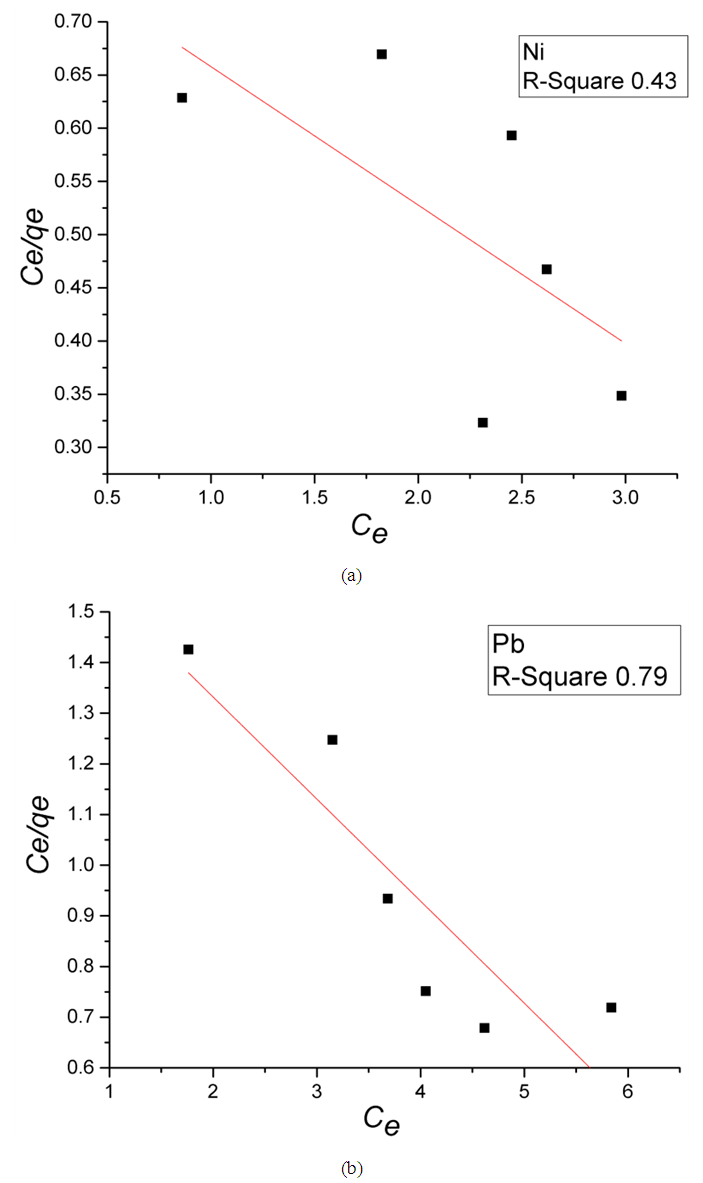Figure 7. Langmuir plots for the adsorption of (a) Ni(II) and (b) Pb(II) on Zn-Al-NTA adsorbent; (t=60 min, pH=5, T=25°C, V=30 ml, m=0.20 g, Co=50 mg/L)
##### 3.5.2. Temkin Isotherm
The Temkin isotherm model suggests that the heat of adsorption of all adsorbate molecules would decrease linearly with the increase in coverage of the adsorbent surface due to adsorbate/adsorbent interactions. The model also proposes that adsorption is characterized by a uniform distribution of binding energies, up to a maximum binding energy. The linear form is expressed as :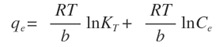Where,
R is the universal constant (8.314 J/K.mol);
T is the absolute temperature (K);
b is a constant related to the heat of adsorption;
KT is the equilibrium binding constant corresponding to the maximum bending energy (L/mg).
Plotting (qe) versus (lnCe) results in a straight line of slope RT/b and intercept (RT ln KT)/b (Figure 8). It can be seen from the Figure that the low correlation coefficient values R2 of 0.67 and 0.88 for Ni and Pb, respectively, are indicative that the experimental data as a whole are not best fitted to Temkin isotherm.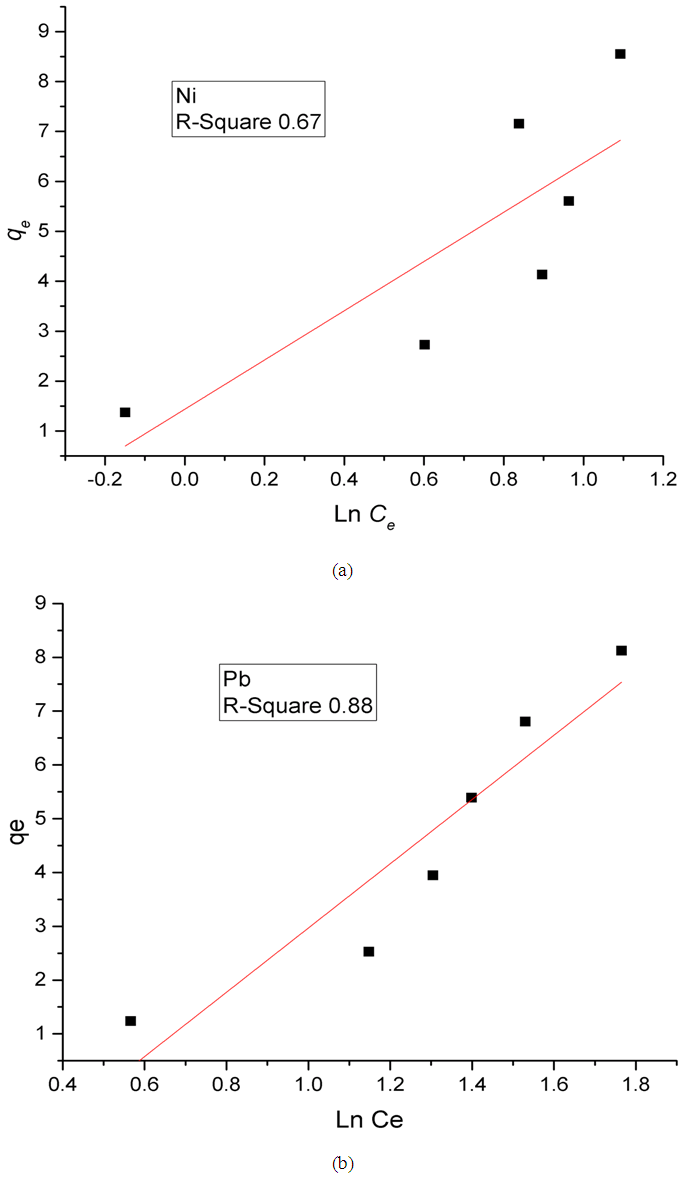Figure 8. Temkin plots for the adsorption of (a) Ni(II) and (b) Pb(II) on Zn-Al-NTA adsorbent; (t=60 min, pH=5, T=25°C, V=30 ml, m=0.20 g, Co=50 mg/L)
##### 3.5.3. Freundlich Isotherm
Freundlich isotherm model assumes a non-ideal adsorption on heterogeneous surfaces in a multilayer coverage. It suggests that stronger binding sites are occupied first, followed by weaker binding sites. In other words, as the degree of site occupation increases, the binding strength decreases. The linear form is shown as :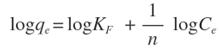Where,
KF is the Freundlich constant related to adsorption capacity (mg/g);
n is the heterogeneity coefficient (indicates how favorable an adsorption process is (g/L).
Plotting (log qe) versus (log Ce) results in a straight line of slope (1/n) and intercept (log KF) (Figure 9). As shown in the Figure, the correlation coefficient values R2 (0.86 and 0.96 for Ni and Pb, respectively) are much closer to one as compared to the results from the Langmuir and Temkin isotherm models, suggesting that the adsorption of Ni(II) and Pb(II) on Zn-Al-NTA is mainly a physical multilayer adsorption that follows Freundlich equation. Correlation coefficient values R2 resulted from fitting the experimental data to Langmuir, Temkin and Freundlich Isotherms are listed in Table 2 along with the parameters of Freundlich isotherm for the adsorption of Ni(II) and Pb(II) on Zn-Al-NTA.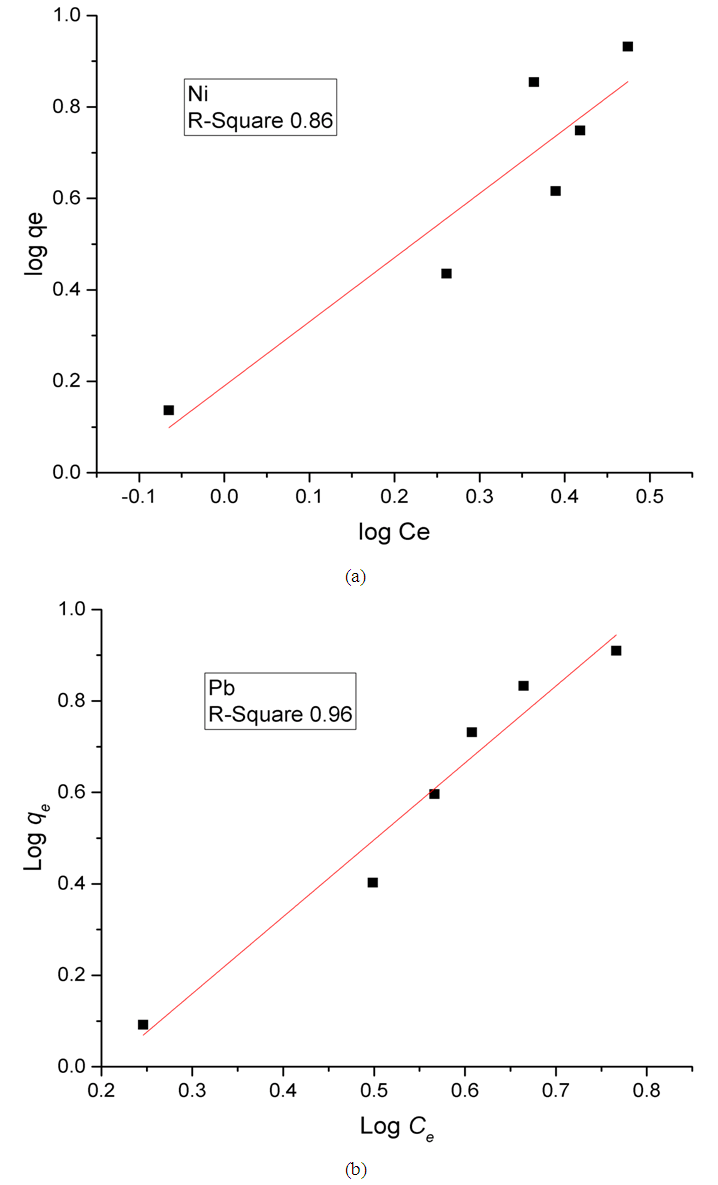Figure 9. Freundlich plots for the adsorption of (a) Ni(II) and (b) Pb(II) on Zn-Al-NTA adsorbent; (t=60 min, pH=5, T=25°C, V=30 ml, m=0.20 g, Co=50 mg/L)
 Table 2. Summary of the correlation coefficient values R2 resulted from fitting the experimental data to Langmuir, Freundlich and Temkin Isotherms along with the parameters of Freundlich isotherm for the adsorption of Ni(II) and Pb(II) on Zn-Al-NTA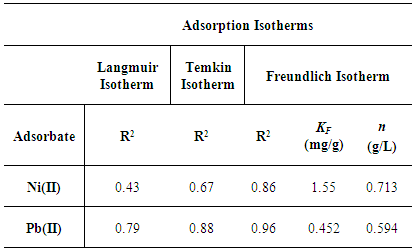The kinetic of adsorption was employed to study the process in which specific amounts of metal ions are diffused from a solution to a boundary layer of the water surrounding Zn-Al-NTA phase. The kinetics of adsorption of Ni(II) and Pb(II) metal ions onto Zn-Al-NTA was conducted by withdrawing and analyzing solution samples at time intervals of 5, 10, 15, 30, 45, 60 and 120 minutes. All kinetic experiments were carried out at optimum adsorption conditions of constant Zn-Al-NTA mass of 0.2 g in 30 mL solution at 25°C and pH 5 with constant initial metal ion concentration of 50 mg/L. The amount of adsorption at time t is calculated using: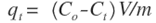Where Co and Ct (mg/L) are the concentrations of the metal ions at the initial time and time t, respectively. V is the volume of the solution (L), and m is the mass of Zn-Al-NTA adsorbent (g). The kinetic data for the adsorption of Ni(II) and Pb(II) metal ions onto Zn-Al-NTA were tested with well-known kinetic models, namely Lagergren’s pseudo first-order model, pseudo second-order model and intra-particle diffusion model. The parameters of these models were calculated and summarized in Table 3.
A) Pseudo first-order kinetics
Pseudo first-order kinetics model  has been most widely used to describe the kinetic process of liquid-solid phase adsorption; i.e., for the adsorption of an adsorbate from an aqueous solution. The linear form of this model is: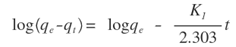Where,
qe is the amount of metal ions adsorbed at equilibrium per unit mass of Zn-Al-NTA (mg/g);
qt is the amount of metal ions adsorbed at time t per unit mass of Zn-Al-NTA (mg/g);
K1 is the rate constant of pseudo first-order adsorption model (mg⋅g−1⋅min−1).
K1 can be evaluated from the graph of log (qe-qt) versus t. Such plot will give a straight line for the pseudo first-order adsorption with (logqe) as intercept and (-K1/2.303) as the slope of the graph (Figure 10).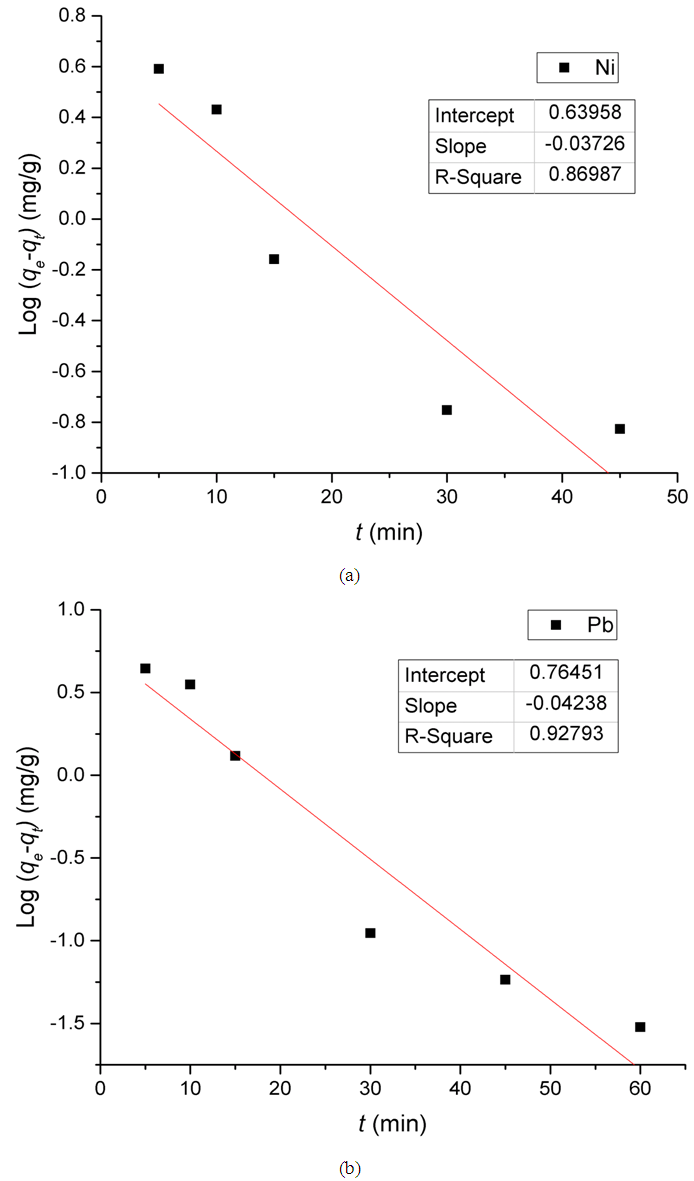Figure 10. Pseudo first-order kinetic model for the adsorption of Ni(II) and Pb(II) metal ions onto Zn-Al-NTA. (pH=5, T=25°C, V=30 ml, m=0.20 g, Co=50 mg/L)
B) Pseudo second-order kinetics
The adsorption kinetics can also be described by pseudo second-order model. The linear form of this model is given by this equation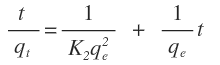Where K2 is the rate constant of pseudo second-order adsorption. If experimental data fit this model, a linear relationship is produced when plotting t/qt versus t, from which K2 and qe can be determined from the slope and intercept from the graph (Figure 11).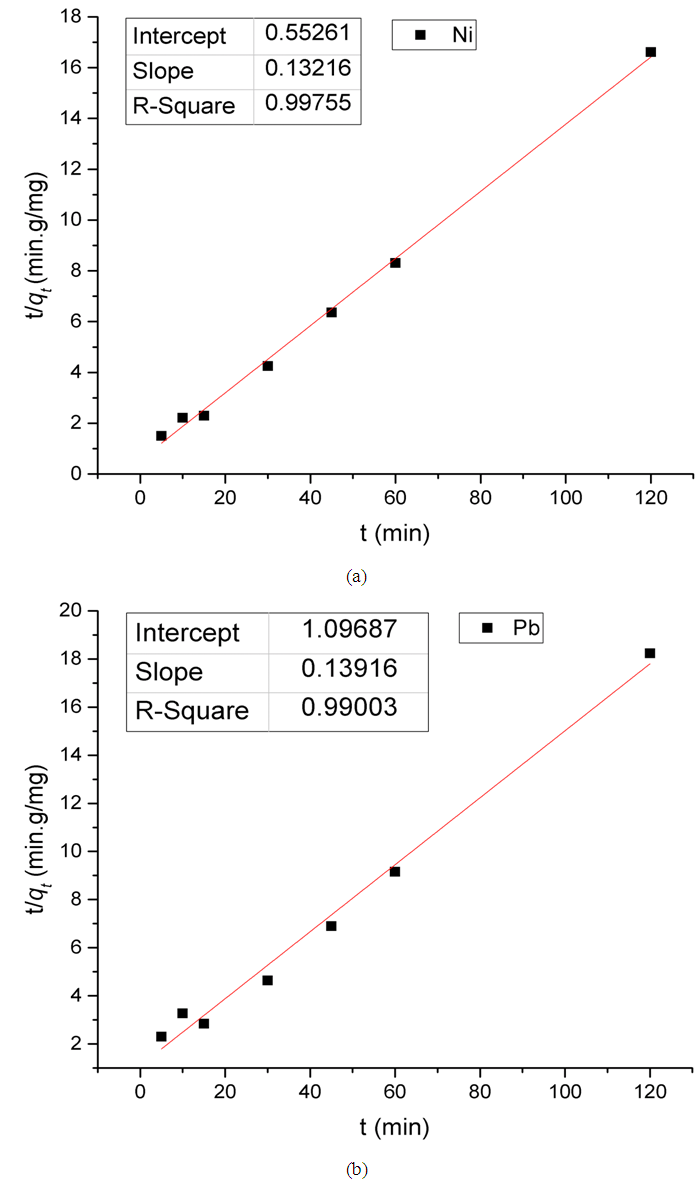Figure 11. Pseudo second-order kinetic model for the adsorption of Ni(II) and Pb(II) on Zn-Al-NTA Composites. (pH=5, T=25°C, V=30 ml, m=0.20 g, Co=50 mg/L)
C) Intra-particle diffusion kinetic model
The intra-particle diffusion model is expressed as: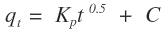Where,
KP is the diffusion rate constant (mg/g.min0.5);
C is a constant that gives an indication on the thickness of the boundary layer (mg/g).
For intra-particle diffusion kinetic model, a plot of qt versus t0.5 gives a linear relationship with constant C as the y-intercept and KP as the slope (Figure 12).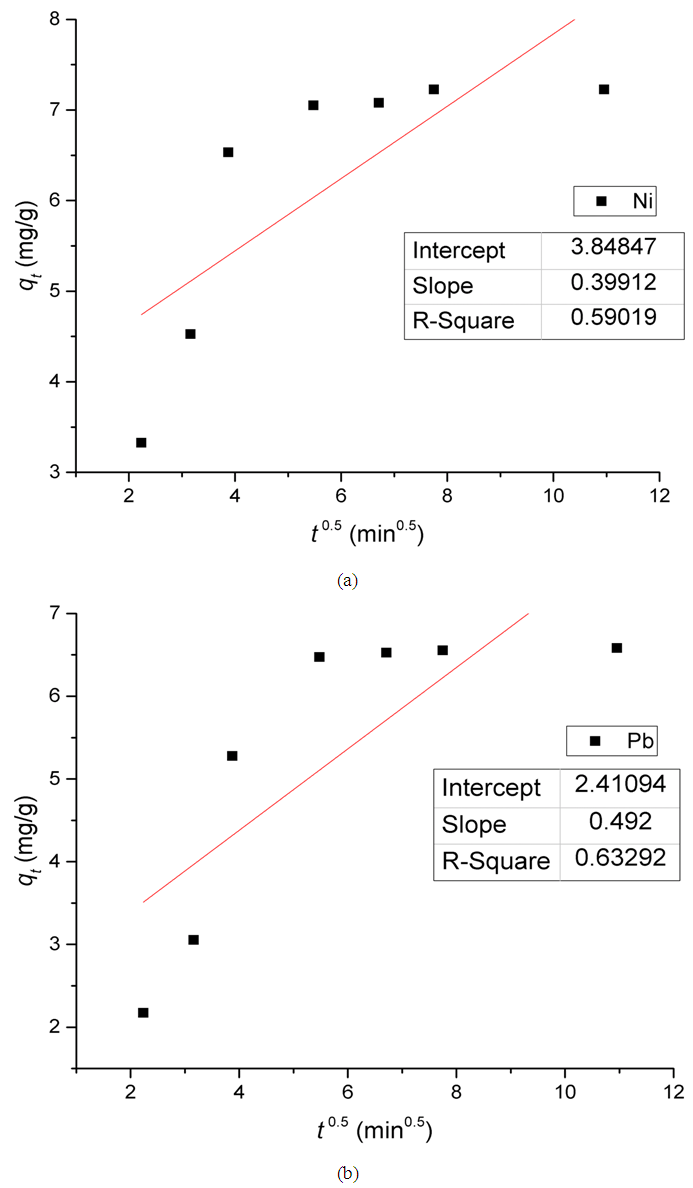Figure 12. Intra-particle diffusion kinetic model for the adsorption of (a) Ni(II) and (b) Pb(II) on Zn-Al-NTA Composites. (pH=5, T=25°C, V=30 ml, m=0.20 g, Co=50 mg/L)
It was found that the calculated parameters of pseudo second-order kinetic model are consistent with the experimental values for both adsorptions of Ni(II) and Pb(II) on Zn-Al-NTA composite (Table 3). For instance, the calculated qe value for adsorption of Ni(II) at 7.567 mg/g is comparable to the experimental value at 7.227 mg/g, unlike the calculated qe value from first-order kinetics at 4.369 mg/g. Additionally, the values for the correlation coefficient of second-order kinetics is close to unity at 0.99755 and 0.99003 for Ni(II) and Pb(II) adsorption, respectively, indicating that the adsorption of Ni(II) and Pb(II) followed the mechanism of second-order kinetics.
 Table 3. The parameters of pseudo first-order, pseudo second-order and intra-particle diffusion kinetic models for the adsorption of Ni(II) and Pb(II) on Zn-Al-NTA composites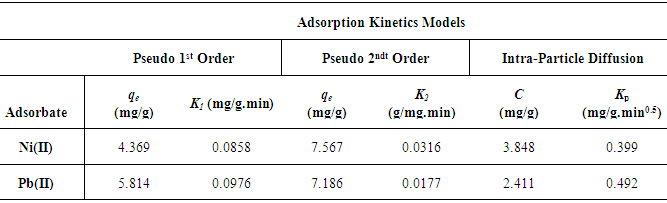### 4. Conclusions

The Zn-Al-LDH material was synthesized and intercalated with NTA successfully. The characterization showed interesting features because it contains numerous number of functional groups or coordination sites within its structure that can easily interact with metal ions onto the surface or in between the layers. It is of interest to convert the anionic synthetic clay into cationic ion-exchange by the simple intercalation reaction of ligand such as NTA. The adsorption process studied and determined by different parameters such as the variation of metal ion concentration of model aqueous solutions, contact time with sorbent, mass of sorbent, and pH of the reaction system. Initial adsorption, first 10 min was very fast, and it reached equilibrium after maximum 60 min. The factors determining the percentage removal of nickel(II) and lead(II) ions onto Zn-Al-NTA was studied, in which the influence of both adsorbent layer surface functional groups of LDH as well as the acetate groups of NTA ligand give the main influence to metal ions adsorption which is mainly a physical multilayer adsorption that follows Freundlich equation. The kinetics study results of the experimental process are relatively well described using the pseudo-second order equation. This is confirmed by the percentage removal of Ni(II) and Pb(II) which could be over 95% in addition to the high coefficient of correlation (R2=0.997) obtained for Pb(II) at the studied concentrations of aqueous solutions. The advantages of Zn-Al-NTA composite material include its availability as low preparation costs, its uses for nano extraction which may be of fundamental importance due to its possible application in the nano purification technology of waste waters.